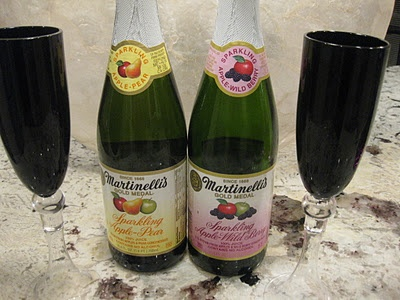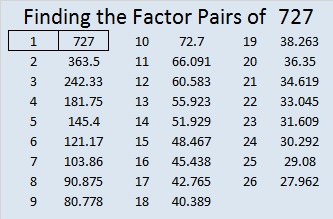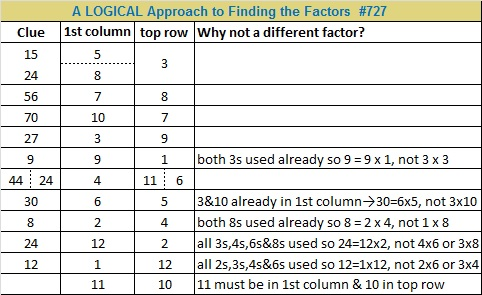# 727 Enjoy Some Sparkling Cider!

Have a happy New Year’s Eve! My husband and I will be enjoying some Sparkling Apple-Pear. I invite you to have some, too.Perhaps the goblet in the puzzle can start off your festivities.Print the puzzles or type the solution on this excel file: 12 Factors 2015-12-28

—————————————————————————————————

• 727 is a prime number.
• Prime factorization: 727 is prime.
• The exponent of prime number 727 is 1. Adding 1 to that exponent we get (1 + 1) = 2. Therefore 727 has exactly 2 factors.
• Factors of 727: 1, 727
• Factor pairs: 727 = 1 x 727
• 727 has no square factors that allow its square root to be simplified. √727 ≈ 26.9629375.727 is a palindrome in base 10 and one other base:

• 727 BASE 10; note that 7(100) + 2(10) + 7(1) = 727.
• 1B1 BASE 22 (B = 11 base 10); note that 1(22²) + 11(22) + 1(1) = 727.

OEIS.org informs us that 727² = 528529, a number whose digits can be split in half to make two consecutive numbers.

Since 727 is a prime number, there is only one way it can be expressed as the sum of consecutive numbers: 363 + 364 = 727.

—————————————————————————————————